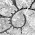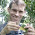## Tuesday, February 12, 2013

### Fix to fastAnc

A colleague recently reported bad behavior in the fast ancestral character estimation function fastAnc that he hypothesized was due to the fact that I extracted the total number of tips in the tree using the shorthand N<-length(tree\$tip) in place of N<-tree\$tip.label. In principle this shouldn't be a problem because list elements, unlike variables, can be called by any unambiguous abbreviation of the element name (that is, if we use the \$ operator - see below).

So, for instance:
> exampleList<-list(x=c(1,2),test=c("A","B","C"))
> exampleList\$t
 "A" "B" "C"
> exampleList\$test2<-c("D","E","F")
> exampleList\$t # no longer umambiguous
NULL
> exampleList\$test
 "A" "B" "C"

As an aside, if we want to call elements in a list accepting only an exact match the name, then we have to use [[ instead of \$. So, for instance:
> exampleList\$test2<-NULL
> exampleList\$t
 "A" "B" "C"
> exampleList[["t"]]
NULL
> exampleList[["test"]]
 "A" "B" "C"
> # tell R to allow partial match
> exampleList[["t",exact=FALSE]]
 "A" "B" "C"

This is the reason why either:
> tree<-pbtree(n=112)
> length(tree\$tip)
 112
> # or
> length(tree\$tip.label)
 112
will, generally speaking, serve equally well in computing the number of tips in a tree.

However, what the exercise above inadvertently shows is that if we were to create a custom "phylo" object (which we have every right to do), we could do so by adding an additional list component, for instance \$tip.states. If we did, it could create confusion when we compute the number of terminal species in the tree using length(tree\$tip). So, for instance:
> x<-fastBM(tree)
> tree\$tip.states<-x
> length(tree\$tip)
 0
> length(tree\$tip.label)
 112

This suggests that it might not be the best programming practice to assume that length(tree\$tip) will invariably compute the number of species in the tree. I have updated fastAnc, here, but I know for a fact that this kind of code shorthand exists in other functions of the phytools package as well. I will try to update these as time goes on.

BTW - in that example with the custom element \$tip.states, here is what fastAnc does:
> fastAnc(tree,x)
Error in root(btree, node = i) :
incorrect node#: should be greater than the number of taxa
> # load the updated source
> source("fastAnc.R")
> fastAnc(tree,x)
113         114         115  ...
-0.59341281 -0.03688321  0.89743347  ...

1.2.